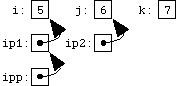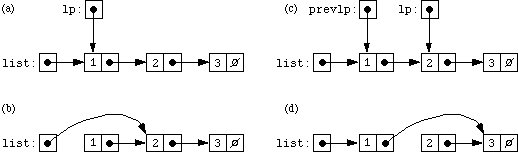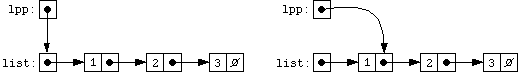# Chapter 22: Pointers to Pointers

Since we can have pointers to int, and pointers to char, and pointers to any structures we've defined, and in fact pointers to any type in C, it shouldn't come as too much of a surprise that we can have pointers to other pointers. If we're used to thinking about simple pointers, and to keeping clear in our minds the distinction between the pointer itself and what it points to, we should be able to think about pointers to pointers, too, although we'll now have to distinguish between the pointer, what it points to, and what the pointer that it points to points to. (And, of course, we might also end up with pointers to pointers to pointers, or pointers to pointers to pointers to pointers, although these rapidly become too esoteric to have any practical use.)

The declaration of a pointer-to-pointer looks like

```	int **ipp;
```
where the two asterisks indicate that two levels of pointers are involved.

Starting off with the familiar, uninspiring, kindergarten-style examples, we can demonstrate the use of ipp by declaring some pointers for it to point to and some ints for those pointers to point to:

```	int i = 5, j = 6; k = 7;
int *ip1 = &i, *ip2 = &j;
```
Now we can set
```	ipp = &ip1;
```
and ipp points to ip1 which points to i. *ipp is ip1, and **ipp is i, or 5. We can illustrate the situation, with our familiar box-and-arrow notation, like this:If we say
```	*ipp = ip2;
```
we've changed the pointer pointed to by ipp (that is, ip1) to contain a copy of ip2, so that it (ip1) now points at j:If we say
```	*ipp = &k;
```
we've changed the pointer pointed to by ipp (that is, ip1 again) to point to k:What are pointers to pointers good for, in practice? One use is returning pointers from functions, via pointer arguments rather than as the formal return value. To explain this, let's first step back and consider the case of returning a simple type, such as int, from a function via a pointer argument. If we write the function

```	f(int *ip)
{
*ip = 5;
}
```
and then call it like this:
```	int i;
f(&i);
```
then f will ``return'' the value 5 by writing it to the location specified by the pointer passed by the caller; in this case, to the caller's variable i. A function might ``return'' values in this way if it had multiple things to return, since a function can only have one formal return value (that is, it can only return one value via the return statement.) The important thing to notice is that for the function to return a value of type int, it used a parameter of type pointer-to-int.

Now, suppose that a function wants to return a pointer in this way. The corresponding parameter will then have to be a pointer to a pointer. For example, here is a little function which tries to allocate memory for a string of length n, and which returns zero (``false'') if it fails and 1 (nonzero, or ``true'') if it succeeds, returning the actual pointer to the allocated memory via a pointer:

```	#include <stdlib.h>

int allocstr(int len, char **retptr)
{
char *p = malloc(len + 1);	/* +1 for \0 */
if(p == NULL)
return 0;
*retptr = p;
return 1;
}
```
The caller can then do something like
```	char *string = "Hello, world!";
char *copystr;
if(allocstr(strlen(string), &copystr))
strcpy(copystr, string);
else	fprintf(stderr, "out of memory\n");
```
(This is a fairly crude example; the allocstr function is not terribly useful. It would have been just about as easy for the caller to call malloc directly. A different, and more useful, approach to writing a ``wrapper'' function around malloc is exemplified by the chkmalloc function we've been using.)

One side point about pointers to pointers and memory allocation: although the void * type, as returned by malloc, is a ``generic pointer,'' suitable for assigning to or from pointers of any type, the hypothetical type void ** is not a ``generic pointer to pointer.'' Our allocstr example can only be used for allocating pointers to char. It would not be possible to use a function which returned generic pointers indirectly via a void ** pointer, because when you tried to use it, for example by declaring and calling

```	double *dptr;
if(!hypotheticalwrapperfunc(100, sizeof(double), &dptr))
fprintf(stderr, "out of memory\n");
```
you would not be passing a void **, but rather a double **.

Another good use for pointers to pointers is in dynamically allocated, simulated multidimensional arrays, which we'll discuss in the next chapter.

As a final example, let's look at how pointers to pointers can be used to eliminate a nuisance we've had when trying to insert and delete items in linked lists. For simplicity, we'll consider lists of integers, built using this structure:

```	struct list
{
int item;
struct list *next;
};
```
Suppose we're trying to write some code to delete a given integer from a list. The straightforward solution looks like this:
```	/* delete node containing i from list pointed to by lp */

struct list *lp, *prevlp;
for(lp = list; lp != NULL; lp = lp->next)
{
if(lp->item == i)
{
if(lp == list)
list = lp->next;
else	prevlp->next = lp->next;
break;
}
prevlp = lp;
}
}
```
This code works, but it has two blemishes. One is that it has to use an extra variable to keep track of the node one behind the one it's looking at, and the other is that it has to use an extra test to special-case the situation in which the node being deleted is at the head of the list. Both of these problems arise because the deletion of a node from the list involves modifying the previous pointer to point to the next node (that is, the node before the deleted node to point to the one following). But, depending on whether the node being deleted is the first node in the list or not, the pointer that needs modifying is either the pointer that points to the head of the list, or the next pointer in the previous node.

To illustrate this, suppose that we have the list (1, 2, 3) and we're trying to delete the element 1. After we've found the element 1, lp points to its node, which just happens to be the same node that the main list pointer points to, as illustrated in (a) below:To remove element 1 from the list, then, we must adjust the main list pointer so that it points to 2's node, the new head of the list (as shown in (b)). If we were trying to delete node 2, on the other hand (as illustrated in (c) above), we'd have to adjust node 1's next pointer to point to 3. The prevlp pointer keeps track of the previous node we were looking at, since (at other than the first node in the list) that's the node whose next pointer will need adjusting. (Notice that if we were to delete node 3, we would copy its next pointer over to 2, but since 3's next pointer is the null pointer, copying it to node 2 would make node 2 the end of the list, as desired.)

We can write another version of the list-deletion code, which is (in some ways, at least) much cleaner, by using a pointer to a pointer to a struct list. This pointer will point at the pointer which points at the node we're looking at; it will either point at the head pointer or at the next pointer of the node we looked at last time. Since this pointer points at the pointer that points at the node we're looking at (got that?), it points at the pointer which we need to modify if the node we're looking at is the node we're deleting. Let's see how the code looks:

```	struct list **lpp;
for(lpp = &list; *lpp != NULL; lpp = &(*lpp)->next)
{
if((*lpp)->item == i)
{
*lpp = (*lpp)->next;
break;
}
}
}
```
That single line
```	*lpp = (*lpp)->next;
```
updates the correct pointer, to splice the node it refers to out of the list, regardless of whether the pointer being updated is the head pointer or one of the next pointers. (Of course, the payoff is not absolute, because the use of a pointer to a pointer to a struct list leads to an algorithm which might not be nearly as obvious at first glance.)

To illustrate the use of the pointer-to-pointer lpp graphically, here are two more figures illustrating the situation just before deleting node 1 (on the left) or node 2 (on the right).In both cases, lpp points at a struct node pointer which points at the node to be deleted. In both cases, the pointer pointed to by lpp (that is, the pointer *lpp) is the pointer that needs to be updated. In both cases, the new pointer (the pointer that *lpp is to be updated to) is the next pointer of the node being deleted, which is always (*lpp)->next.

One other aspect of the code deserves mention. The expression

```	(*lpp)->next
```
describes the next pointer of the struct node which is pointed to by *lpp, that is, which is pointed to by the pointer which is pointed to by lpp. The expression
```	lpp = &(*lpp)->next
```
sets lpp to point to the next field of the struct list pointed to by *lpp. In both cases, the parentheses around *lpp are needed because the precedence of * is lower than ->.

As a second, related example, here is a piece of code for inserting a new node into a list, in its proper order. This code uses a pointer-to-pointer-to-struct list for the same reason, namely, so that it doesn't have to worry about treating the beginning of the list specially.

```	/* insert node newlp into list */

struct list **lpp;
for(lpp = &list; *lpp != NULL; lpp = &(*lpp)->next)
{
struct list *lp = *lpp;
if(newlp->item < lp->item)
{
newlp->next = lp;
*lpp = newlp;
break;
}
}
}
```

Read sequentially: prev next up top